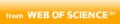Autor(en)
Shareef, Zeeshan; Trächtler, Ansgar
Titel
Simultaneous path planning and trajectory optimization for robotic manipulators using discrete mechanics and optimal control
Teil von
• Robotica, 2016-06, Vol.34 (6), p.1322-1334
Ort / Verlag
Cambridge, UK: Cambridge University Press
Quelle
Web of ScienceBeschreibungen
In robotics, path planning and trajectory optimization are usually performed separately to optimize the path from the given starting point to the ending point in the presence of obstacles. In this paper, path planning and trajectory optimization for robotic manipulators are solved simultaneously by a newly developed methodology called Discrete Mechanics and Optimal Control (DMOC). In DMOC, the Lagrange–d'Alembert equation is discretized directly unlike the conventional variational optimization method in which first the Euler–Lagrange equations are derived and then discretization takes place. In this newly developed method, the constraints for optimization of a desired objective function are the forced discrete Euler–Lagrange equations. In this paper, DMOC is used for simultaneous path planning and trajectory optimization for robotic manipulators in the presence of known static obstacles. Two numerical examples, applied on a DELTA parallel robot, are discussed to show the applicability of this new methodology. The optimal results obtained from DMOC are compared with the other state-of-the-art techniques. The difficulties and problems associated in using the DMOC for Parallel Kinematic Machine (PKM) are also discussed in this paper.
Format
Sprache(n)
Englisch
Identifikator(en)
ISSN: 0263-5747
ISSN: 1469-8668
DOI: 10.1017/S0263574714002318